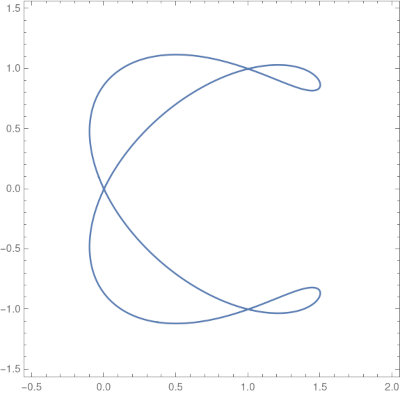# Three interesting curves

Here are three curves that have interesting names and interesting shapes.

## The fish curve

The fish curve has parameterization

x(t) = cos(t) – sin²(t)/√2
y(t) = cos(t) sin(t)

We can plot this curve in Mathematica with

```    ParametricPlot[
{Cos[t] - Sin[t]^2/Sqrt, Cos[t] Sin[t]},
{t, 0, 2 Pi}]
```

to get the following.It’s interesting that the image has two sharp cusps: the parameterization consists of two smooth functions, but the resulting image is not smooth. That’s because the velocity of the curve is zero at 3π/4 and 7π/4.

I found the fish curve by poking around Mathematica’s `PlainCurveData` function. I found the next two in the book Mathematical Models by H. Martyn Cundy and A. P. Rollett.

## The electric motor curve

Next is the “electric motor” curve. Cundy and Rollett’s book doesn’t provide illustrations for the plane curves it lists on pages 71 and 72, and I plotted the electric motor curve because I was curious why it was called that.

The curve has equation

y²(y² – 96) = x² (x² – 100)

and we can plot it in Mathematica with

```    ContourPlot[ y^2 (y^2 - 96) == x^2 (x^2 - 100),
{x, -20, 20}, {y, -20, 20}```

which gives the following.Sure enough, it looks like the inside of an electric motor!

## The ampersand curve

I was also curious about the “ampersand curve” because of its name. The curve has equation

(y² – x²) (x – 1) (2x – 3) = 4 (x² + y² – 2x

and can be plotted in Mathematica with

```    ContourPlot[(y^2 - x^2) (x - 1) (2 x - 3) ==
4 (x^2 + y^2 - 2 x)^2,
{x, -0.5, 2}, {y, -1.5, 1.5},
PlotPoints -> 100]
```

The produces the following pleasant image.Note that the code specifies `PlotPoints`. Without this argument, the default plotting resolution produces a misleading plot, making it appear that the curve has three components.